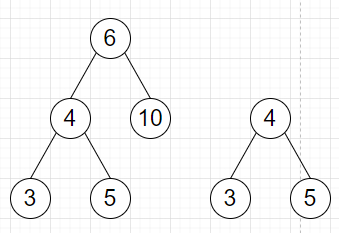# Program to check whether one tree is subtree of other or not in Python

Suppose we have two binary trees. We have to check whether second tree is a subtree of first one or not.

So, if the input is likethen the output will be True.

To solve this, we will follow these steps −

• Define a function solve() . This will take root, target

• if root is null and target is also null, then

• return True

• if root is null or target is null, then

• return False

• if value of root is same as value of target, then

• return solve(left of root, left of target) and solve(right of root, right of target)

• otherwise,

• return solve(left of root, target) or solve(right of root, target)

Let us see the following implementation to get better understanding −

## Example

Live Demo

class TreeNode:
def __init__(self, data, left = None, right = None):
self.val = data
self.left = left
self.right = right
class Solution:
def solve(self, root, target):
if root == None and target == None:
return True
if root == None or target == None:
return False
if root.val == target.val:
return self.solve(root.left, target.left) and
self.solve(root.right, target.right)
else:
return self.solve(root.left, target) or
self.solve(root.right, target)
ob = Solution()
root1 = TreeNode(6)
root1.left = TreeNode(4)
root1.right = TreeNode(10)
root1.left.left = TreeNode(3)
root1.left.right = TreeNode(5)
root2 = TreeNode(4)
root2.left = TreeNode(3)
root2.right = TreeNode(5)
print(ob.solve(root1, root2))

## Input

root1 = TreeNode(6)
root1.left = TreeNode(4)
root1.right = TreeNode(10)
root1.left.left = TreeNode(3)
root1.left.right = TreeNode(5)
root2 = TreeNode(4)
root2.left = TreeNode(3)
root2.right = TreeNode(5)

## Output

True

Updated on: 21-Oct-2020

194 Views